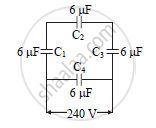Share

# A Network of Four Capacitors of 6 μF Each is Connected to a 240 V Supply. Determine the Charge on Each Capacitor. - Physics

#### Question

A network of four capacitors of 6 μF each is connected to a 240 V supply. Determine the charge on each capacitor.#### Solution

C1,C2 and C3 are in series

:.1/C_s=1/C_1+1/C_2+1/C_3

=1/(6xx10^(-6))+1/(6xx10^(-6))+1/(6xx10^(-6))

1/C_s=3/(6xx10^(-6))

∴ Cs = 2x10-6 F

Cs and C4 are parallel

∴ equivalent resistance

C = Cp = Cs + C4

= 2x10-6 + 6x10-6

C = 8x10-6

∴v = v1+ v2+ v3

240 = 3v1  {v1=v2=v3}

∴ v1  80 volt

Also charge on C1,C2 ,C3 are same.

Q1 = Q2 = Q3 = Q

Q = C1v1

= 6x10-6 x 80

Q = 480x10-6C and Q4 = 6x10-6x 240= 144 × 10-5

Q4 = 1.44 × 10-3 C

Is there an error in this question or solution?

#### APPEARS IN

2013-2014 (March) (with solutions)
Question 6.4 | 3.00 marks
Solution A Network of Four Capacitors of 6 μF Each is Connected to a 240 V Supply. Determine the Charge on Each Capacitor. Concept: Condensers in Series and Parallel,.
S